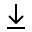MathBin Diophantine Equation Member

A Diophantine Equation is a polynomial equation, usually in two or more unknowns, such that only the integer solutions are sought or studied (an integer solution is such that all the unknowns take integer values). A linear Diophantine equation equates the sum of two or more monomials, each of degree 1 in one of the variables, to a constant. An exponential Diophantine equation is one in which exponents on terms can be unknowns.

Diophantine problems have fewer equations than unknown variables and involve finding integers that work correctly for all equations. In more technical language, they define an algebraic curve, algebraic surface, or more general object, and ask about the lattice points on it.

The word Diophantine refers to the Hellenistic mathematician of the 3rd century, Diophantus of Alexandria, who made a study of such equations and was one of the first mathematicians to introduce symbolism into algebra. The mathematical study of Diophantine problems that Diophantus initiated is now called Diophantine analysis.

While individual equations present a kind of puzzle and have been considered throughout history, the formulation of general theories of Diophantine equations (beyond the theory of quadratic forms) was an achievement of the twentieth century.

1. Coins in a Jar (JS)
2. Farm Animals (JS)
3. Pirate's Treasure (JS)
4. Twenty Bank Deposits (JS)